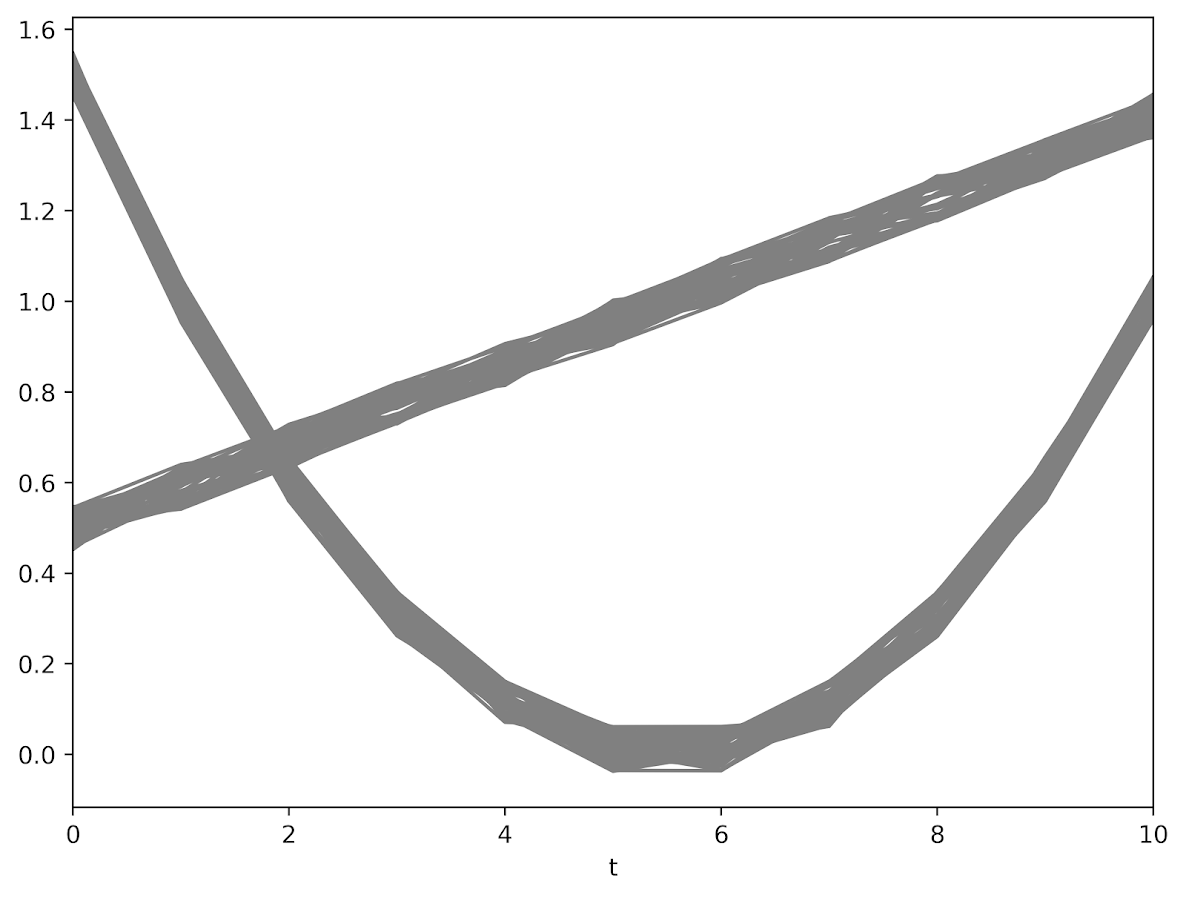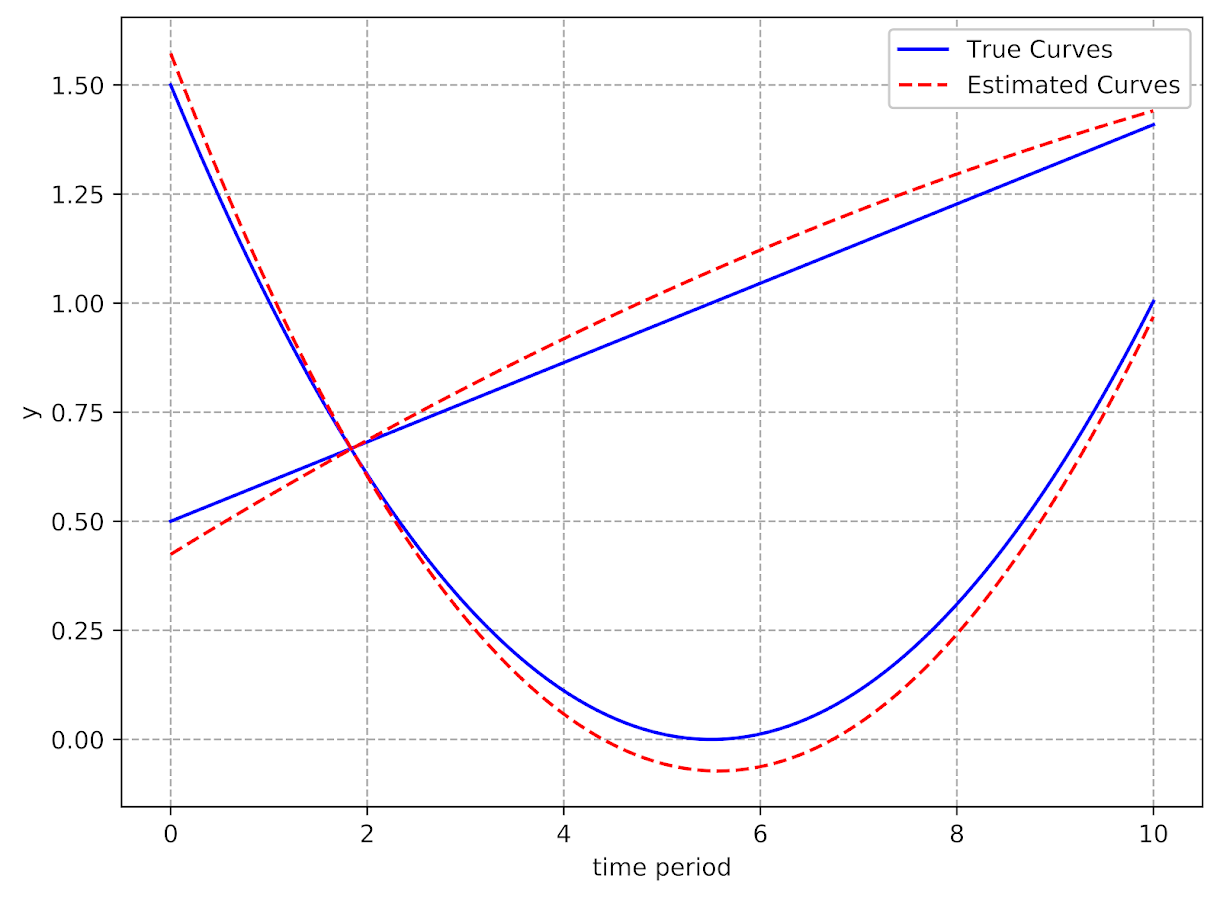# Using pytorch to estimate group based traj models

Deep learning, tensors, pytorch. Now that I have that seo junk out of the way 🙂 – I’ve been trying to teach myself some “Deep Learning”, as it is what all of the cool kids are doing these days.

I was having a hard time though with many of the different examples. Many are for image data, and so it was hard for me to translate that to actual applications I am interested in. Many do talk about dimension reduction and reducing to hidden layers, so I thought that was similar in nature to latent class analysis, such as group-based-trajectory-modelling (GBTM).

If you aren’t familiar with GBTM, imagine a scenario in which you cluster data, and then you estimate a different regression model to predict some outcome for each subset of the clustered data. This is just a way to do that whole set-up in one go, instead of doing each part separately. It has quite a few different names – latent class analysis and mixture modelling are two common ones. The only thing really different about GBTM is that you have repeated observations – so if you follow the same person over time, they should always be assigned to the same cluster/mixture.

In short you totally can do GBTM models in deep learning libraries (as I will show), but actually most examples that I have walked through are more akin to dimension reduction of columns (so like PCA/Canonical Correlation). But the deep learning libraries are flexible enough to do the latent class analysis I want here. As far as I can tell they are basically just a nice way to estimate systems of equations (with a ton of potential parameters, and do it on the GPU if you want).

So I took it as a challenge to estimate GBTM models in a deep learning library – here pytorch. In terms of the different architectures/libraries (e.g. pytorch, tensorflow, Vowpal Wabbit) I just chose pytorch because one of my co-workers suggested pytorch was easier to learn!

I’ve posted a more detailed notebook of the code, but it worked out quite well. So first I simulated two groups of data (50 observations in each group and 11 time periods). I added a tiny bit of random noise, so this (I was hoping) should be a pretty tame problem for the machine to learn.The code to generate a pytorch module and have the machine churn out the gradients is pretty slick (less than 30 lines total of non-comments). Many GBTM code bases make you do the analysis in wide format (so one row is an observation), but here I was able to figure out how to set it up in long data format, which makes it real easy to generalize to unbalanced data.It took quite a few iterations to converge though, (iterations were super fast, but it is a tiny problem, so not sure how timing will generalize) and only converged when using the Adam optimizer (stochastic gradient descent converged to an answer with a similar mean square error, but not to anywhere near the right answer). These models are notorious for converging to sub-optimal locations, so that may just be an intrinsic part of the problem and a good library needs to do better with starting conditions.

I have a few notes about potential updates to the code at the end of my Jupyter notebook. For count or binomial 0/1 data, that should be a pretty easy update. Also need to write code to do out of sample predictions (which I think I can figure out as well). A harder problem I am not sure how to figure out is to do an equation for the latent groups inside of the function. And I don’t know how to get standard errors for the coefficient estimates. Hopefully I can figure that out while trying to teach myself some more deep learning. I have a few convolution ideas I want to try out for spatial-temporal crime forecasting and include proactive police feedback, but I won’t get around to them for quite awhile I imagine.

1./  May 14, 2020

Nice work!
is there any python toolkit (not the estimate like the one here) for group based traj models that you know of? I have seen crimeCV in R, but I am looking for python

•#### apwheele

/  May 14, 2020

No not that I am aware of. I did notice just today that the tslearn library had several for k-means, https://tslearn.readthedocs.io/en/stable/gen_modules/tslearn.clustering.html#module-tslearn.clustering (I prefer these traj models though for the examples I have worked with).

•/  May 14, 2020

•#### kathy

/  March 16, 2021

Hi, I am also looking for group based traj tools in python. Did you find anything after that?

•#### apwheele

/  March 16, 2021

No I am not familiar with any other libraries offhand Kathy. Typically what I search for are ‘mixture model’, as that seems to be a bit more common terminology than ‘latent classes’. The biggest issue is generalized linear functions, and that since group traj is with repeated data the mixture should be at the group level.

So maybe it is possible to parameterize this in say pymc3, but it would take a bit of work (at least for me) to figure that out.

•#### kathy

/  March 16, 2021